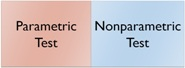## What are Parametric Tests & Non-Parametric Tests?Parametric Tests & Non-Parametric Tests
Parametric Tests (for example, “t” test, and “f” test) are regarded as one of the most powerful tests. It is used during the stage of data analysis of a research work.

Parametric Tests are used when certain basic assumptions are fulfilled. If those assumptions are not fulfilled, that kind of tests comes under the ambit of Non-Parametric Tests (such as, chi square test, and Mann-Whitney Test).
Following are the basic assumptions of the parametric tests. If these assumptions are fulfilled, then parametric tests could be used; otherwise, non-parametric tests would be employed. Let’s discuss the basic assumptions of:

1.    Normality of Distribution: The sample (which has been drawn from the population) must be normally distributed for parametric tests. If samples are not normally distributed, it would not fall under the category of non-parametric tests; that is why, non-parametric tests are also known as distribution-free tests.

2. Randomization: The condition for selecting sample from the populations must be random. It means any technique, which is under the category of probability sampling technique, needs to be adopted. If samples are not selected through the process of randomization, we cannot apply parametric tests; in that case, we would apply non-parametric tests.

3. Homogeneity of Variance: The samples have equal, or nearly equal, variances. Homogeneity means sameness (in other words, more or less it should be same). For example, if we calculate the variance of two populations from where samples are drawn, they must have more or less same variance.  If there is a wide difference among the variance of the sample, parametric tests cannot be used; in such case, non-parametric tests will be used.

4.   Null-Hypothesis: This assumption is pre-requisite for both parametric as well as non-parametric tests. Therefore, this assumption is not the distinctive feature of parametric tests; this is the common feature of both parametric and non-parametric tests.
In both type of tests (parametric test or non-parametric test), a null-hypothesis must be formed. A null-hypothesis states that there is no significant difference or relationship between two or more parameters.

What are Parametric and Non-Parametric Data?

There are two types of data which are recognized during the application of statistical treatments. These are the following:

i. Parametric Data: It refers to the data which are measured. As it has already been discussed above, parametric tests assume that the data are normally (or nearly normally) distributed. Parametric tests are applied to both interval and ratio scaled data.
ii.Non-Parametric Data: Data of this type are either counted (nominal) or ranked (ordinal).